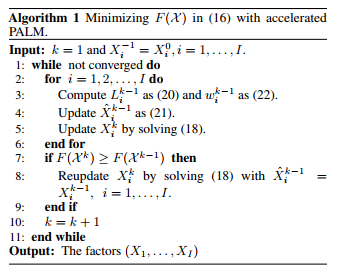# A Unified Convex Surrogate for the Schatten-p Norm### Abstract

The Schatten-p norm (0 < p < 1) has been widely used to replace the nuclear norm for better approximating the rank function. However, existing methods are either 1) not scalable for large scale problems due to relying on singular value decomposition (SVD) in every iteration, or 2) specific to some p values, e.g., 1/2, and 2/3. In this paper, we show that for any p, p1, and p2 > 0 satisfying 1/p = 1/p1 + 1/p2, there is an equivalence between the Schatten-p norm of one matrix and the Schatten-p1 and the Schatten-p2 norms of its two factor matrices. We further extend the equivalence to multiple factor matrices and show that all the factor norms can be convex and smooth for any p > 0. In contrast, the original Schatten-p norm for 0 < p < 1 is non-convex and non-smooth. As an example we conduct experiments on matrix completion. To utilize the convexity of the factor matrix norms, we adopt the accelerated proximal alternating linearized minimization algorithm and establish its sequence convergence. Experiments on both synthetic and real datasets exhibit its superior performance over the state-of-the-art methods. Its speed is also highly competitive.

Type
Publication
AAAI Conference on Artificial Intelligence